# lfd.registration package¶

## Class inheritance diagram¶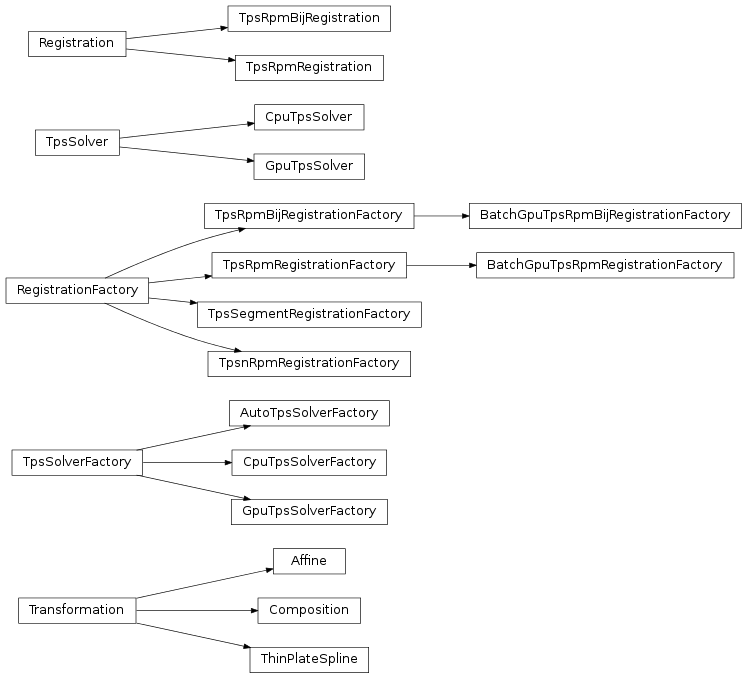## lfd.registration.plotting_openrave module¶

lfd.registration.plotting_openrave.registration_plot_cb(sim, x_nd, y_md, f)[source]

## lfd.registration.registration module¶

class lfd.registration.registration.BatchGpuTpsRpmBijRegistrationFactory(demos, actionfile=None, n_iter=20, em_iter=1, reg_init=0.1, reg_final=0.0001, rad_init=0.01, rad_final=0.0001, rot_reg=<Mock id='140724509947856'>, outlierprior=0.1, outlierfrac=0.01, prior_fn=None, f_solver_factory=<lfd.registration.solver.GpuTpsSolverFactory object at 0x7ffcfa59c190>, g_solver_factory=<lfd.registration.solver.GpuTpsSolverFactory object at 0x7ffcfa59c210>)[source]

Similar to TpsRpmBijRegistrationFactory but batch_register and batch_cost are computed in batch using the GPU

batch_cost(test_scene_state)[source]
batch_register(test_scene_state)[source]
class lfd.registration.registration.BatchGpuTpsRpmRegistrationFactory(demos)[source]

Similar to TpsRpmRegistrationFactory but batch_register and batch_cost are computed in batch using the GPU

batch_cost(test_scene_state)[source]
batch_register(test_scene_state)[source]
cost(demo, test_scene_state)[source]
register(demo, test_scene_state, callback=None)[source]
class lfd.registration.registration.Registration(demo, test_scene_state, f, corr)[source]

Bases: object

get_objective()[source]
class lfd.registration.registration.RegistrationFactory(demos=None)[source]

Bases: object

batch_cost(test_scene_state)[source]

Gets costs of every demonstration scene in demos registered onto the test scene

Returns: A dict that maps from the demonstration names that are in demos to the numpy.array of partial cost
batch_register(test_scene_state, callback=None)[source]

Registers every demonstration scene in demos onto the test scene

Returns: A dict that maps from the demonstration names that are in demos to the Registration

Note

Derived classes might ignore the argument callback

cost(demo, test_scene_state)[source]

Gets costs of registering the demonstration scene onto the test scene

Parameters: demo – Demonstration which has the demonstration scene test_scene_state – SceneState of the test scene A 1-dimensional numpy.array containing the partial costs used for the registration; the sum of these is the objective used for the registration. The exact definition of these partial costs is given by the derived classes.
register(demo, test_scene_state, callback=None)[source]

Registers demonstration scene onto the test scene

Parameters: demo – Demonstration which has the demonstration scene test_scene_state – SceneState of the test scene callback – callback function; the derived classes define the arguments of the functoin A Registration
class lfd.registration.registration.TpsRpmBijRegistration(demo, test_scene_state, f, g, corr, rad)[source]
get_objective()[source]
static get_objective2(x_nd, y_md, f, g, corr_nm, rad)[source]

Returns the following 10 objectives:

•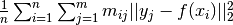•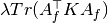••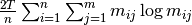•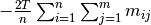•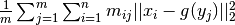•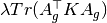••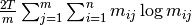•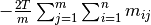class lfd.registration.registration.TpsRpmBijRegistrationFactory(demos=None, n_iter=20, em_iter=1, reg_init=0.1, reg_final=0.0001, rad_init=0.01, rad_final=0.0001, rot_reg=<Mock id='140724509947856'>, outlierprior=0.1, outlierfrac=0.01, prior_fn=None, f_solver_factory=<lfd.registration.solver.GpuTpsSolverFactory object at 0x7ffcfa58ffd0>, g_solver_factory=<lfd.registration.solver.GpuTpsSolverFactory object at 0x7ffcfa59c090>)[source]

As in:

1. Schulman, J. Ho, C. Lee, and P. Abbeel, “Learning from Demonstrations through the Use of Non-Rigid Registration,” in Proceedings of the 16th International Symposium on Robotics Research (ISRR), 2013.

Tries to solve the optimization problem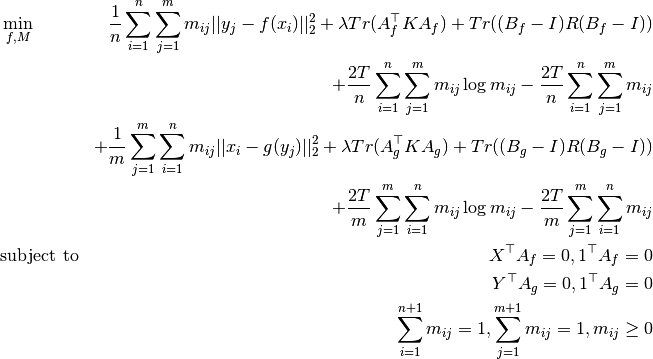cost(demo, test_scene_state)[source]

Gets the costs of the forward and backward thin plate spline objective of the resulting registration

Parameters: demo – Demonstration which has the demonstration scene test_scene_state – SceneState of the test scene A 1-dimensional numpy.array containing the residual, bending and rotation cost of the forward and backward spline, each already premultiplied by the respective coefficients.
register(demo, test_scene_state, callback=None)[source]
class lfd.registration.registration.TpsRpmRegistration(demo, test_scene_state, f, corr, rad)[source]
get_objective()[source]
static get_objective2(x_nd, y_md, f, corr_nm, rad)[source]

Returns the following 5 objectives:

••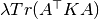•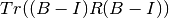••class lfd.registration.registration.TpsRpmRegistrationFactory(demos=None, n_iter=20, em_iter=1, reg_init=0.1, reg_final=0.0001, rad_init=0.01, rad_final=0.0001, rot_reg=<Mock id='140724509947856'>, outlierprior=0.1, outlierfrac=0.01, prior_fn=None, f_solver_factory=<lfd.registration.solver.GpuTpsSolverFactory object at 0x7ffcfa58ff10>)[source]

As in:

1. Chui and A. Rangarajan, “A new point matching algorithm for non-rigid registration,” Computer Vision and Image Understanding, vol. 89, no. 2, pp. 114-141, 2003.

Tries to solve the optimization problem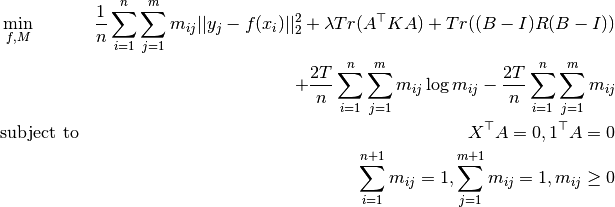cost(demo, test_scene_state)[source]

Gets the costs of the thin plate spline objective of the resulting registration

Parameters: demo – Demonstration which has the demonstration scene test_scene_state – SceneState of the test scene A 1-dimensional numpy.array containing the residual, bending and rotation cost, each already premultiplied by the respective coefficients.
register(demo, test_scene_state, callback=None)[source]
class lfd.registration.registration.TpsSegmentRegistrationFactory(demos)[source]
batch_cost(test_scene_state)[source]
batch_register(test_scene_state)[source]
cost(demo, test_scene_state)[source]
register(demo, test_scene_state, callback=None)[source]
class lfd.registration.registration.TpsnRpmRegistrationFactory(demos)[source]

TPS-RPM using normals information

batch_cost(test_scene_state)[source]
batch_register(test_scene_state)[source]
cost(demo, test_scene_state)[source]
register(demo, test_scene_state, callback=None)[source]

## lfd.registration.settings module¶

lfd.registration.settings.BEND_COEF_DIGITS = 6
lfd.registration.settings.EM_ITER = 1

number of inner iterations for RPM algorithms

lfd.registration.settings.MAX_CLD_SIZE = 150
lfd.registration.settings.N_ITER = 20

number of outer iterations for RPM algorithms

lfd.registration.settings.OURLIER_FRAC = 0.01
lfd.registration.settings.OUTLIER_CUTOFF = 0.01
lfd.registration.settings.OUTLIER_PRIOR = 0.1
lfd.registration.settings.RAD = (0.01, 0.0001)

initial and final temperature for RPM algorithms

lfd.registration.settings.REG = (0.1, 0.0001)

initial and final smoothing regularizer coefficient for RPM algorithms

lfd.registration.settings.ROT_REG

rotation regularizer coefficients

## lfd.registration.solver module¶

class lfd.registration.solver.AutoTpsSolverFactory(use_cache=True, cachedir=None)[source]
class lfd.registration.solver.CpuTpsSolver(N, QN, NKN, NRN, NR, x_nd, K_nn, rot_coef)[source]
solve(wt_n, y_nd, bend_coef, f_res)[source]
class lfd.registration.solver.CpuTpsSolverFactory(use_cache=True, cachedir=None)[source]
get_solver(x_nd, rot_coef)[source]
get_solver_mats(x_nd, rot_coef)[source]
class lfd.registration.solver.GpuTpsSolver(N, QN, NKN, NRN, NR, x_nd, K_nn, rot_coef)[source]
solve(wt_n, y_nd, bend_coef, f_res)[source]
class lfd.registration.solver.GpuTpsSolverFactory(use_cache=True, cachedir=None)[source]
get_solver(x_nd, rot_coef)[source]
get_solver_mats(x_nd, rot_coef)[source]
class lfd.registration.solver.TpsSolver(N, QN, NKN, NRN, NR, x_nd, K_nn, rot_coef)[source]

Bases: object

Fits thin plate spline to data using precomputed matrix products

solve(wt_n, y_nd, bend_coef, f_res)[source]
class lfd.registration.solver.TpsSolverFactory(use_cache=True, cachedir=None)[source]

Bases: object

get_solver(x_nd, rot_coef)[source]
get_solver_mats(x_nd, rot_coef)[source]

Precomputes several of the matrix products needed to fit a TPS exactly. A TPS is fit by solving the system: N’(Q’WQ + b K + R)N z = -N’(Q’W’y + N’R) x = Nz where K and R are padded with zeros appropriately.

Returns: N, QN, N’KN, N’RN N’R

## lfd.registration.tps module¶

Functions for fitting and applying thin plate spline transformations

class lfd.registration.tps.ThinPlateSpline(d=3)[source]
x_na

centers of basis functions

w_ng

weights of basis functions

lin_ag

transpose of linear part, so you take x_na.dot(lin_ag)

trans_g

translation part

compute_jacobian(x_ma)[source]
static create_from_optimization(x_na, y_ng, bend_coef, rot_coef, wt_n)[source]

Solves the optimization problem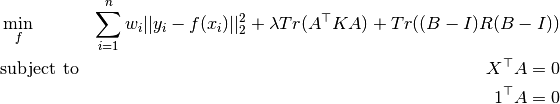Parameters: x_na – source cloud,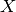y_ng – target cloud,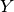bend_coef – smoothing, penalize non-affine part,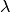rot_coef – angular_spring, penalize rotation,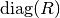wt_n – weight the points,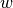A ThinPlateSpline f
get_objective()[source]

Returns the following 3 objectives:

•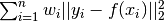••Note

Implementation covers general case where there is a wt_n and bend_coef per dimension

transform_points(x_ma)[source]
update(x_na, y_ng, bend_coef, rot_coef, wt_n, theta, N=None, z=None)[source]
lfd.registration.tps.balance_matrix3(*args, **kwargs)[source]
lfd.registration.tps.balance_matrix3_cpu(prob_nm, max_iter, row_priors, col_priors, outlierfrac, r_N=None)[source]

Balances matrix, including the prior row and column.

Example

>>> from lfd.registration.tps import balance_matrix3_cpu
>>> import numpy as np
>>> n, m = (100, 150)
>>> prob_nm = np.random.random((n,m))
>>> p_n = 0.1 * np.random.random(n)
>>> p_m = 0.1 * np.random.random(m)
>>> outlierfrac = 1e-2
>>> prob_nm0 = balance_matrix3_cpu(prob_nm, 10, p_n, p_m, outlierfrac)
>>> prob_NM = np.empty((n+1, m+1))
>>> prob_NM[:n, :m] = prob_nm.copy()
>>> prob_NM[:n, m] = p_n.copy()
>>> prob_NM[n, :m] = p_m.copy()
>>> prob_NM[n, m] = np.sqrt(np.sum(p_n)*np.sum(p_m))
>>> a_N = np.r_[np.ones(n), m*outlierfrac]
>>> b_M = np.r_[np.ones(m), n*outlierfrac]
>>> for _ in xrange(10):
...     prob_NM = prob_NM / (prob_NM.sum(axis=0) / b_M)[None, :]
...     prob_NM = prob_NM / (prob_NM.sum(axis=1) / a_N)[:, None]
...
>>> prob_nm1 = prob_NM[:n,:m]
>>> np.allclose(prob_nm0, prob_nm1)
True

lfd.registration.tps.balance_matrix3_gpu(prob_nm, max_iter, row_priors, col_priors, outlierfrac, r_N=None)[source]
lfd.registration.tps.balance_matrix4(prob_nm, max_iter, p_n, p_m)[source]

Like balance_matrix3 but doesn’t normalize the p_m row and the p_n column

Example

>>> from lfd.registration.tps import balance_matrix4
>>> import numpy as np
>>> n, m = (100, 150)
>>> prob_nm = np.random.random((n,m))
>>> p_n = 0.1 * np.random.random(n)
>>> p_m = 0.1 * np.random.random(m)
>>> prob_nm0 = balance_matrix4(prob_nm, 10, p_n, p_m)
>>> prob_nm1 = prob_nm.copy()
>>> for _ in xrange(10):
...     prob_nm1 = prob_nm1 / (prob_nm1.sum(axis=0) + p_m)[None, :]
...     prob_nm1 = prob_nm1 / (prob_nm1.sum(axis=1) + p_n)[:, None]
...
>>> np.allclose(prob_nm0, prob_nm1)
True

lfd.registration.tps.loglinspace(start, stop, num)[source]

Return numbers spaced with a constant ratio.

Returns num numbers in the interval [start, stop], with constant ratio between consecutive numbers.

Parameters: start – The starting value of the sequence. stop – The end value of the sequence. num – Number of samples to generate.

Note

Unlike np.linspace, a singleton sequence with stop is returned when num is 1.

Example

>>> loglinspace(1.0, 100.0, 3)
array([   1.,   10.,  100.])
>>> loglinspace(10.0, 0.001, 5)
array([  1.00000000e+01,   1.00000000e+00,   1.00000000e-01,
1.00000000e-02,   1.00000000e-03])
>>> loglinspace(2, 4, 1)
array([ 4.])

lfd.registration.tps.nan2zero(x)[source]
lfd.registration.tps.prepare_fit_ThinPlateSpline(x_nd, y_md, corr_nm, fwd=True)[source]

Takes into account outlier source points and normalization of points

lfd.registration.tps.solve_eqp1(H, f, A, ret_factorization=False)[source]

solve equality-constrained qp min .5 tr(x’Hx) + tr(f’x) s.t. Ax = 0

lfd.registration.tps.tps_apply_kernel(distmat, dim)[source]
if d=2:
k(r) = 4 * r^2 log(r)
d=3:
k(r) = -r

import numpy as np, scipy.spatial.distance as ssd x = np.random.rand(100,2) d=ssd.squareform(ssd.pdist(x)) print np.clip(np.linalg.eigvalsh( 4 * d**2 * log(d+1e-9) ),0,inf).mean() print np.clip(np.linalg.eigvalsh(-d),0,inf).mean()

Note the actual coefficients (from http://www.geometrictools.com/Documentation/ThinPlateSplines.pdf) d=2: 1/(8*sqrt(pi)) = 0.070523697943469535 d=3: gamma(-.5)/(16*pi**1.5) = -0.039284682964880184

lfd.registration.tps.tps_eval(x_ma, lin_ag, trans_g, w_ng, x_na)[source]
lfd.registration.tps.tps_fit3(x_na, y_ng, bend_coef, rot_coef, wt_n, ret_factorization=False)[source]
lfd.registration.tps.tps_grad(x_ma, lin_ag, _trans_g, w_ng, x_na)[source]
lfd.registration.tps.tps_kernel_matrix(x_na)[source]
lfd.registration.tps.tps_kernel_matrix2(x_na, y_ma)[source]
lfd.registration.tps.tps_rpm(x_nd, y_md, f_solver_factory=None, n_iter=20, em_iter=1, reg_init=0.1, reg_final=0.0001, rad_init=0.01, rad_final=0.0001, rot_reg=<Mock id='140724509947856'>, outlierprior=0.1, outlierfrac=0.01, prior_prob_nm=None, callback=None)[source]
lfd.registration.tps.tps_rpm_bij(x_nd, y_md, f_solver_factory=None, g_solver_factory=None, n_iter=20, em_iter=1, reg_init=0.1, reg_final=0.0001, rad_init=0.01, rad_final=0.0001, rot_reg=<Mock id='140724509947856'>, outlierprior=0.1, outlierfrac=0.01, prior_prob_nm=None, callback=None)[source]

## lfd.registration.transformation module¶

Register point clouds to each other

arrays are named like name_abc abc are subscripts and indicate the what that tensor index refers to

index name conventions:
m: test point index n: training point index a: input coordinate g: output coordinate d: gripper coordinate
class lfd.registration.transformation.Affine(lin_ag, trans_g)[source]
compute_jacobian(x_ma)[source]
transform_points(x_ma)[source]
class lfd.registration.transformation.Composition(fs)[source]
compute_jacobian(x_ma)[source]
transform_points(x_ma)[source]
class lfd.registration.transformation.Transformation[source]

Bases: object

Object oriented interface for transformations R^d -> R^d

compute_jacobian(x_ma)[source]
compute_numerical_jacobian(x_d, epsilon=0.0001)[source]

numerical jacobian

transform_bases(x_ma, rot_mad, orthogonalize=True, orth_method='cross')[source]

orthogonalize: none, svd, qr

transform_hmats(hmat_mAD)[source]

Transform (D+1) x (D+1) homogenius matrices

transform_points(x_ma)[source]
transform_vectors(x_ma, v_ma)[source]
lfd.registration.transformation.orthogonalize3_cross(mats_n33)[source]

turns each matrix into a rotation

lfd.registration.transformation.orthogonalize3_qr(_x_k33)[source]
lfd.registration.transformation.orthogonalize3_svd(x_k33)[source]